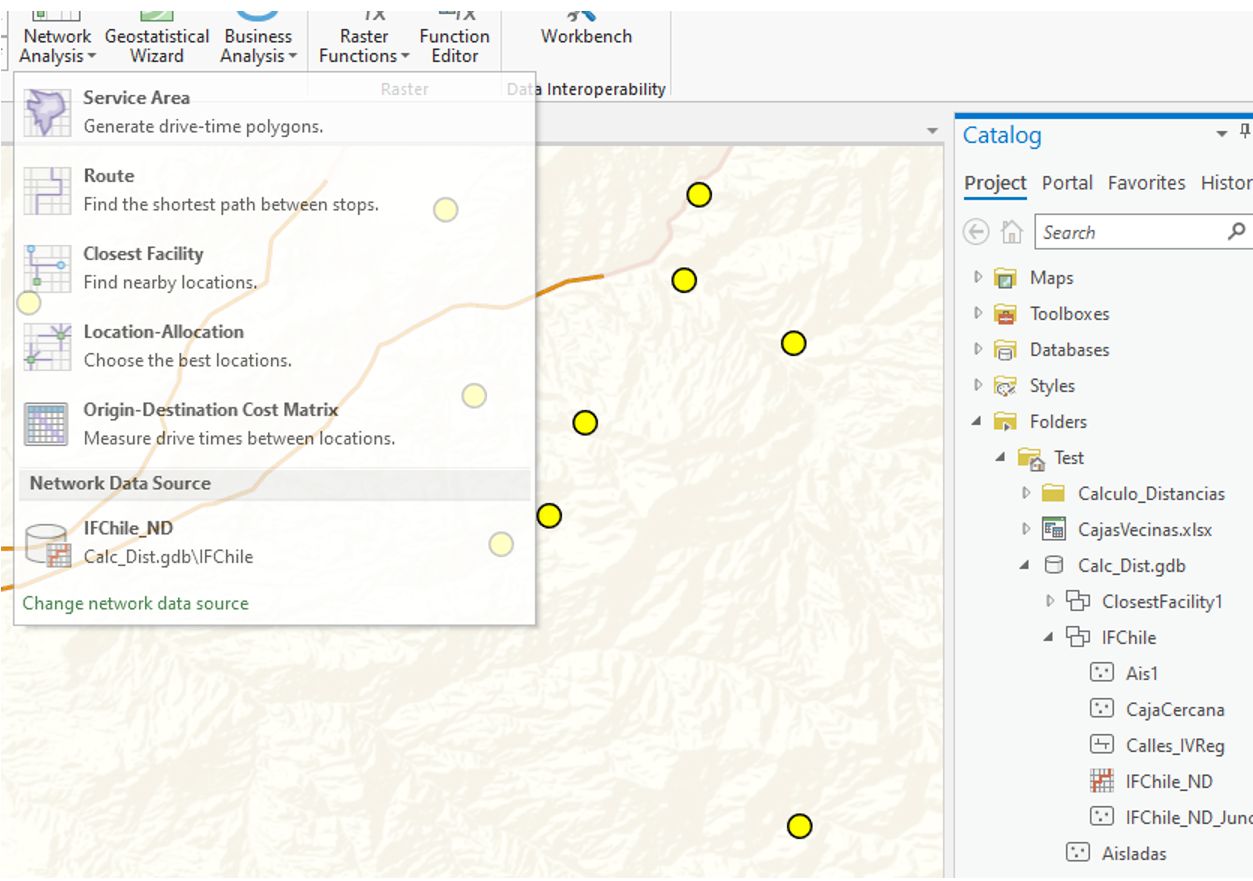# Calculate Distance Manhattan through Streets

224
1
08-13-2019 03:11 PM
Highlighted
byEsri Contributor

Hi Community

I have an important challenge. I need to calculate distance (Manhattan) around a network of streets, in order to join points from isolated areas, to points of interest to the community. The idea is to be able to calculate the distance between these isolated community points, to these points of interest and that ArcGIS, calculate what is the best point of interest that the person should reach, by shorter distance, by calculating the Manhattan distance. A quick analysis is by means of the "near" toolbar, but it only calculates the euclidean distance which is not real for the study.I have performed tests using network dataset, but it does not satisfy what the study requires.
What I thought was to calculate a proximity table using the "Generate Near Table" and then toolbar, extract the junction points as the "From_X, From_Y" and "Near_X, Near_Y" layer and calculate the distance between them using the street axes , through network analyst, specifically using the "Closest Facility" tool but it didn't work. This also does not necessarily prove to be the shortest distance in Manhattan, because it will depend on the road axes.Do you have any idea how to proceed with this issue within ArcGIS Pro or ArcMap?

by